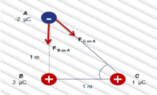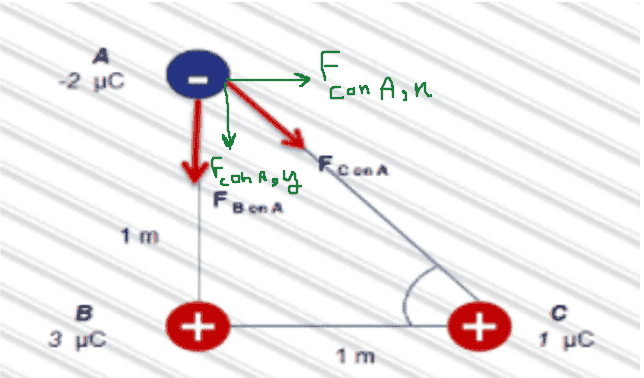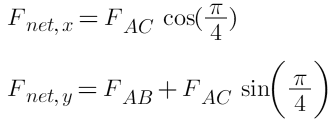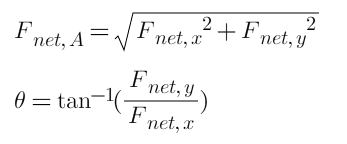# Net Force of A: Diagram Analysis

• Spotify

#### Spotify

Homework Statement
Find the net force of A as shown in the diagram.
Relevant Equations
FAB=KqAqB/r2
Fx=Fcosθ
Fy=Fsinθ
FR=square root of the total of Fx^2+the total of Fy^2
θ=tan-1(Fy/Fx)
Find the net force of A as shown in the diagram.

#### Attachments

•solve.PNG
8 KB · Views: 33## \ ##

I think you want to find Fnet A ...Tell me which part you need explanation.

•berkeman
Homework Statement:: Find the net force of A as shown in the diagram.
Relevant Equations:: FAB=KqAqB/r2
Fx=Fcosθ
Fy=Fsinθ
FR=square root of the total of Fx^2+the total of Fy^2
θ=tan-1(Fy/Fx)

Find the net force of A as shown in the diagram.
You need to find the vector forces ## F_{AB} ## and ## F_{AC} ## and sum those force vectors to get the total force on A. Can you show us your work on that?

•MatinSAR